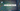July 18, 2019A number consists of digits. Example: 843. Its a 3-digit number. Radix sort, start by sorting numbers first by their most significant digit, then next, and so on.

So, in simpler terms. If there are maximum n numbers, and max d-digits in a number. This algorithm runs on array d times, and sort the numbers according to their current place digit.

To sort the numbers based on a digit, the any sorting technique can be used. But, most importantly the sort must be a stable sort.If you look at the pseudo code (assuming we have n numbers, and all are d-digits long):

``````for (int i=0; i<d; i++;) {
//Sort the array on digit place-i
}``````

See the code here (explanation is below code):

``````public class RadixSort {
private int[] arr;

this.arr = arr;
}

/**
* Using CountSort as stable sort
*/
private void countSort(int position) {
int[] c = new int;  //0 to 9
for (int i=0; i<this.arr.length; i++) {
int digit = this.getDigit(this.arr[i], position);
c[digit] ++;
}
//accumulate
for (int i=1; i<c.length; i++) {
c[i] = c[i] + c[i-1];
}

int[] res = new int[this.arr.length];
for (int i=this.arr.length-1; i>=0; i--) {
int digit = this.getDigit(this.arr[i], position);

res[c[digit]-1] = this.arr[i];
c[digit] --;
}

this.arr = res;
System.out.println(ArrayUtils.toString(arr));
}

private int getDigit(int num, int position) {
return num/position % 10;
}

/**
* Main method for radix sort
*/
public void sort() {
int max = ArrayUtils.getMaxValue(this.arr);

int position = 1;

while (max/position > 0) {
this.countSort(position);

position *= 10;
}
}
}``````

## Explanation

• First, we need to get the maximum number from the array. Since, Radix algorithm will run as long as the maximum number of digits in array.
• In method countSort(), we are using count sort as helper sort algorithm. But, the catch here is that we need to sort the array based on the digit position. We are passing the position of digit.
• We need a way to fetch the digit from the number. Note, we are passing the position
``````Example number: 839
Objective: We need 9, then 3, then 8

To get remainder, we need modulus (%) of a number by 10. We will get the last digit. But, how to get the any other digit?
See method: getDigit()

We first need to reduce the number by dividing with position. And, then take modulus with 10.

i.e. num/position % 10;``````
• Lets get back to countSort()
• As you have seen Count Sort
• First, where we prepare array-c, to get the number of occurrences of a number. We modified that logic by taking that digit defined by position passed.
• Then, we place number according the position.
• And, we repeat this algorithm untill number of digits of maximum numbers finished.

## Key Points

• It is not a comparison sort.
• It uses a stable sort as a helper sort method.
• This sort the number according to the position of digits.
• It is used for relatively smaller set of numbers.

## Runtime complexity

The algorithm runs on time complexity of O(d(n + k)) ~= O(n) in worst/average case. Note: Count sort took O(n + k) time, this takes d times the count sort time.

## Why Stable Sort is required as secondary sort here

Since, the radix algorithm works on digit-by-digit basis. Consider two numbers:

``````18
14``````

So, during first round, the order will become:

``````14
18``````

In second round, where we will be comparing most significant digit. If our algorithm is not stable, then any unstable algorithm might put the order like:

``````18
14``````

Which is wrong, Right!